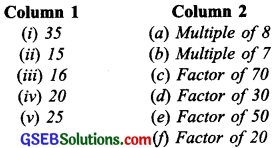# GSEB Solutions Class 6 Maths Chapter 3 Playing With Numbers Ex 3.1

Gujarat Board GSEB Textbook Solutions Class 6 Maths Chapter 3 Playing With Numbers Ex 3.1 Textbook Questions and Answers.

## Gujarat Board Textbook Solutions Class 6 Maths Chapter 3 Playing With Numbers Ex 3.1

Question 1.
Write all the factors of the following numbers:
(a) 24
(b) 15
(c) 21
(d) 27
(e) 12
(f) 20
(g) 18
(h) 23
(i) 36
Solution:
(a) 24
Since, 24 = 1 x 24
24 = 2 x 12
24 = 3 x 8
24 = 4 x 6
All the factors of 24 are: 1, 2, 3, 4, 6, 8, 12 and 24.

(b) 15
Since, 15 = 1 x 15
15 = 3 x 5
All the factors of 15 are: 1, 3, 5 and 15.(c) 21
Since, 21 = 1 x 21
21 = 3 x 7
All the factors of 21 are: 1, 3, 7 and 21.

(d) 27
Since, 27 = 1 x 27
27 = 3 x 9
All the factors of 27 are: 1, 3, 9 and 27.

(e) 12
Since, 12 = 1 x 12
12 = 2 x 6
12 = 3 x 4
All the factors of 12 are: 1, 2, 3, 4, 6 and 12.

(f) 20
Since, 20 = 1 x 20
20 = 2 x 10
20 = 4 x 5
All the factors of 20 are: 1, 2, 4, 5, 10 and 20.(g) 18
Since, 18 = 1 x 18
18 = 2 x 9
18 = 3 x 6
The factors of 18 are: 1, 2, 3, 6, 9 and 18.

(h) 23
Since, 23 = 1 x 23
All the factors of 23 are: 1 and 23.

(i) 36
Since, 36 = 1 x 36
36 = 2 x 18
36 =3 x 12
36 = 49
36 = 6 x 6
All the factors of 36 are: 1, 2, 3, 4, 6, 9, 12, 18 and 36.Question 2.
Write first five multiples of:
(a) 5
(b) 8
(c) 9
Solution:
(a) 5
Since, 5 x 1 = 5
5 x 2 = 10
5 x 3 = 15
5 x 4 = 20
5 x 5 = 25
The first five multiples of 5 are: 5, 10, 15, 20 and 25.

(b) 8
Since, 8 x 1 = 8
8 x 2 = 16
8 x 3 = 24
8 x 4 = 32
8 x 5 = 40
The first five multiples of 8 are: 8, 16, 24, 32 and 40.

(c) 9
Since, 9 x 1 = 9
9 x 2 = 18
9 x 3 = 27
9 x 4 = 36
9 x 5 = 45
The first five multiples of 9 are: 9, 18, 27, 36 and 45.Question 3.
Match the items in column 1 with the items in column 2.Solution:
(i) → (b) ( 7 x 5 = 35)
(ii) → (d) (15 x 2 = 30)
(iii) → (a) (8 x 2 = 16)
(iv) → (f) (20 x 1 = 20)
(v) → (e) (25 x 2 = 50)Question 4.
Find all the multiples of 9 upto 100.
Solution:
Since, 9 x 1 = 9
9 x 2 = 18
9 x 3 = 27
9 x 4 = 36
9 x 5 = 45
9 x 6 = 54
9 x 7 = 63
9 x 8 = 72
9 x 9 = 81
9 x 10 = 90
9 x 11 = 99
All the multiples of 9 upto 100 are: 9, 18, 27, 36, 45, 54, 63, 72, 81, 90 and 99.